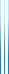# Courses

236646 Constraint Satisfaction Problems Spring 2022

A constraint satisfaction problem consists of a collection of variables and constraints. Each variable has an associated domain. The corresponding problem asks whether there is an assignment to the variables that satisﬁes all constraints; though this is not the only interesting problem which one can ask about a CSP instance. Two standard examples are SAT and $k$-coloring. Related problems are MAX-SAT and MAX-CUT, in which the goal is to maximize the number of satisfied constraints.

In this class, we will study CSPs from several angles:

• Dichotomy theorems: SAT is NP-hard. What happens if we limit the type of constraints one is allowed to use? We will prove Schaefer’s dichotomy theorem, which states that if the variables are Boolean, then each type of CSP is either in P or NP-complete.
• Hardness of approximation: Consider a set of linear equations over $\mathit{GF}(2)$. A random assignment satisfies half of them, and in particular gives a $1/2$ approximation to the problem of maximizing the number of satisfied constraints. Can this be improved? We will prove Håstad’s result, showing that it is NP-hard to improve over this trivial algorithm. We will also show that the Goemans–Williamson algorithm is tight for MAX-CUT, assuming the unique games conjecture (we skipped this part).
• How easy is it to refute a given CNF? We will consider Resolution, the proof system behind SAT solvers. We will prove exponential lower bounds on Resolution refutations of Tseitin contradictions on expanders, and (possibly) on refutations of random 3CNFs with the appropriate density, following classical work of Ben-Sasson and Wigderson.

Time-permitting, we will also briefly consider several other topics: exponential time algorithms for $k$SAT; certifying the unsatisfiability of random $k$CNFs; and the isolation lemma, with applications to approximate counting and sampling.

Lecture notes for the class.

Here are the topics we covered in class:

1. 20 March 2022: Introduction (Sections 1.1–1.3)
2. 27 March 2022: Gadget reductions and two algorithms (Sections 2.1–2.3)
3. 3 April 2022: Polymorphisms and invariants (Sections 2.4–2.5)
4. 10 April 2022: Proof of Schaefer’s theorem (Sections 2.6–2.7)
5. 24 April 2022: Introduction to inapproximability and PCP theorem (Sections 3.1–3.2)
6. 1 May 2022: Parallel repetition, the long code, and introduction to linearity testing (Sections 3.2–3.3)
7. 8 May 2022: Fourier analysis, linearity testing, dictatorship testing, folding (Sections 3.4–3.5)
8. 15 May 2022: Hardness of MAX-3LIN (Section 3.6)
9. 22 May 2022: Resolution (Sections 5.1–5.3)
10. 29 May 2022: Tseitin contradictions (Section 5.4)
11. 7 June 2022 [Tuesday, 14:30, Taub 2]: Short proofs are narrow (Section 5.5)
12. 12 June 2022: Exponential time algorithms for SAT (Section 6)
13. 26 June 2022? [note no class on June 19] (plan): TBD

Assignments:

• Assignment 1, published 11 April 2022, due 1 May 2022 (date flexible). Solution.
• Assignment 2, published 15 May 2022, due 5 June 2022 (date flexible).
• Assignment 3, published 12 June 2022, due 3 July 2022 (date flexible).

Submission is by e-mail. I will post my own solutions once all submissions arrive.